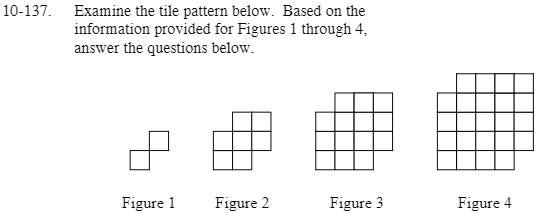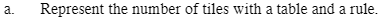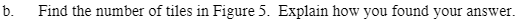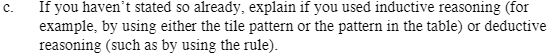### Home > CAAC > Chapter 10 > Lesson 10.4.2 > Problem10-137

10-137.Make a table with the first column as the figure number and the second column as the number of tiles in the figure.

Rule: x2 + 2x − 1There are 34 tiles in figure 5.
Each figure has a square of the figure number + the figure number + one less than the figure number.
There are other possible patterns.